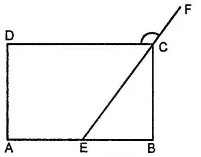Can a quadrilateral ABCD be a parallelogram if

(i) ∠ D + ∠ B = 180°

(ii) AB = DC = 8 cm, AD = 4 cm and BC = 4.4 cm

(iii) ∠ A = 70° and ∠ C = 65°

Asked by Aaryan | 1 year ago |  102

##### Solution :-

(i) For ∠ D + ∠ B = 180°, quadrilateral ABCD may or may not be a parallelogram. Along with this condition, the following conditions should also be fulfilled.

The sum of the measures of adjacent angles should be 180º.

Opposite angles should also be of same measures.

(ii) No. Opposite sides AD and BC are of different lengths.

(iii) No. Opposite angles A and C have different measures.

Answered by Sakshi | 1 year ago

### Related Questions

#### In the given figure, PQRS is a kite. Find the values of x and y.

In the given figure, PQRS is a kite. Find the values of x and y.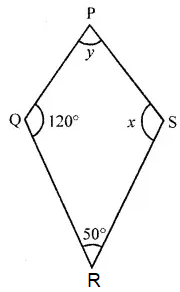#### In the given isosceles trapezium ABCD, ∠C = 102°. Find all the remaining angles of the trapezium.

In the given isosceles trapezium ABCD, ∠C = 102°. Find all the remaining angles of the trapezium.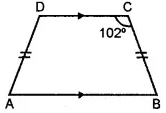#### In the given figure, ABCD is a rhombus and ∠ABD = 50°. Find :

In the given figure, ABCD is a rhombus and ∠ABD = 50°. Find :

(i) ∠CAB

(ii) ∠BCD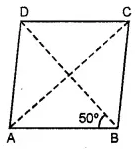#### In the given figure, ABCD is a rectangle and diagonals intersect at O. If ∠AOB = 118°, find

In the given figure, ABCD is a rectangle and diagonals intersect at O. If ∠AOB = 118°, find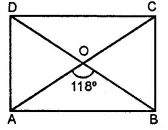(i) ∠ABO

(iii) ∠OCB